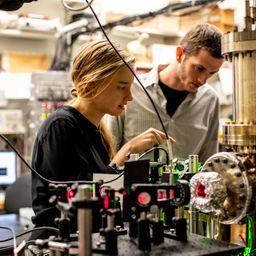## 1.36 c V

Quantum Physics

Atomic Physics

### Discussion

You must be signed in to discuss.
##### Top Physics 103 EducatorsLB##### Marshall S.

University of Washington##### Jared E.

University of Winnipeg

### Video Transcript

So in this problem, we have photons, um, incident on him at all. So there, 450 nanometers. So 4 50 times 10 to the minus nine meters pulls lambda. And we want the most energetic electrons there. Benton in an arc of 20 centimeters. So our cools 0.2 meters and the magnetic field is too 0.0 Times 10 to the minus five. Cussler. Yes, and we want to get the work function. So how do we convert Lett's? Let's think about this information about what information we can kind of extracts from that. We could probably get some kind of energy from that. Um, anything I can find the formulas for particles in a magnetic field, so I don't have to sort of reinvent the wheel, although maybe it would be easier than searching, but I'm gonna take a no pause the video and quickly Look, I have found the formula. I was looking for just a question. 19.5 ours M V over Q b. So I'm gonna go ahead and write that down. Now let's figure out. So we have the mass. We have the charge of the electron. We have to be filled me of the radius. So that means we get the velocities. We think once we get the velocity, then we can go back and say, OK, um, we can determine the kinetic energy and that we can get the work function, so I'm just gonna kind of right This is, um, rearrange. This bee is our Q B right? I am. It's all right. Groups who are a Q B. Yeah, divided by am Great. And so I'm gonna be a true physicist, not evaluate any numbers until the very end. So now I have the velocities and let's think about our kinetic energy work formula. So that tells you that the kinetic that tells us that kinetic energy is the, um, energy of the incident. Photons. So H c over Lambda. That would be the energy of a proton with that lambda, um minus the work function. And so we can go ahead and self. We are going to solve for that so we can say fries equal to K E minus each see derided by Lambda and then kinetic energy. It's gonna be 1/2 on B squared. So then we're square this thing R Q B, but if I am squared minus H C over Lambda. So that's what we should get for the work function. And then we converted TV. But, um, this is kind of take a little bit of time. Something go ahead and possibly while I do this calculation. Okay, so I did my calculations. They came out negative. And then I was like, Oops, I made an error solving for if I so that should actually be a plus. And then this should be a minus. And then the gun, that should be a plus. And this should be a minus. So and then I would that I got in a really small number. And then I converted that TV, and then I got a more reasonable number, which was 1.35 Um, like trump holds.University of Washington

#### Topics

Quantum Physics

Atomic Physics

##### Top Physics 103 EducatorsLB##### Marshall S.

University of Washington##### Jared E.

University of Winnipeg